## Rational Expressions

o        A rational expression is a ratio of polynomials:

Examples: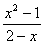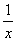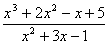### Excluded Values

Whenever an expression containing variables is present in the denominator of a fraction, you should be alert to the possibility that certain values of the variables might make the denominator equal to zero, which is forbidden. This means that when we are talking about rational expressions we can no longer say that the variable represents “any real number.” Certain values may have to be excluded. For example, in the expression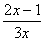,

we cannot allow the value x = 0 so we would parenthetically add the comment (x ¹ 0), and for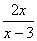we would say (x ¹ 3) . In the case of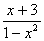we would exclude both x = 1 and x = –1, since either choice would make the denominator zero.

We don’t care if the numerator is zero. If the numerator is zero, that just makes the whole rational expression zero (assuming, of course, that the denominator is not zero), just as with common fractions. Recall that 0/4 = 0, but 4/0 is undefined.

It is important to keep this in mind as you work with rational expressions, because it can happen that you are trying to solve an equation and you get one of the “forbidden” values as a solution. You would have to discard that solution as being unacceptable. You can also get some crazy results if you don’t pay attention to the possibility that the denominator might be zero for certain values of the variable. For example, the celebrated proof that 0 = 1 and other nonsense.

#### Proof that 1 = 0

And other nonsense

Can you identify the flaw in this argument?

Let x = 1. Then

 Given: x = 1 Multiply both sides by x: x2 = x Subtract x from both sides: x2 – x = 0 Factor out an x: x(x – 1) = 0 Divide both sides by (x ‑ 1): x = 0 But x = 1, so substitute 1 for x to get: 1 = 0

This is a very simple variant of this classic ‘proof’. Once you see the trick*, you can construct more elaborate versions that do a better job of concealing the error, and you can vary it to ‘prove’ other nonsense such as 1 = 2.

For example:

Let x = 1 . Then

 Given: x = 1 Multiply both sides by –1: -x = –1 Add x2 to both sides: x2 - x = x2 – 1 Factor both sides: x(x – 1) = (x – 1)(x + 1) Divide both sides by (x – 1): x = (x + 1) Substituting 1 back in for x gives the result: 1 = 2

*The trick:  We are dividing by zero because if x = 1 then (x – 1) = 0. Thus, all of these “proofs” are invalid because they use an illegal step.